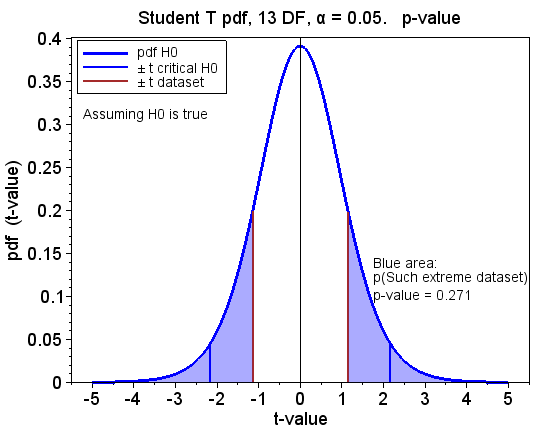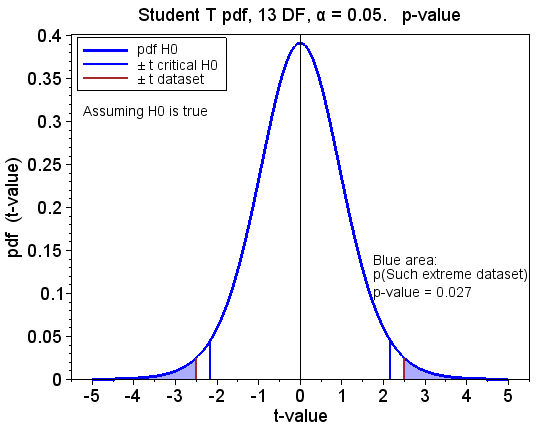## tirsdag 17. juni 2014

### Hypothesis testing of temperature trends

This is the second blog post in a series of five that analyse trends in the global surface temperatures. The posts put emphasis on the mathematics and the statistics used in the analyses. The posts are numbered 1 to 5. They should be read consecutively.

Post 1    Linear regression analysis
Post 2    Hypothesis testing of temperature trends
Post 3    Confidence intervals of temperature trends
Post 4    Statistical power of temperature trends
Post 5    Piecewise linear regression applied to temperature trends

The posts are gathered in this pdf document.

Start of post 2, Hypothesis testing of temperature trends:

The decision of whether a calculated temperature trend is statistically significant or not is based on hypothesis testing. The null hypothesis H0 is that the underlying long term trend is zero and that a calculated trend different from zero is caused by random noise on the measurements. The alternative hypothesis H1 is that the underlying long term trend is different from zero.

The t-value of the trend is its estimated slope [°C/year] divided with its 1-σ uncertainty [°C/year]. It is a dimensionless number. The t-value follows a Student's t-distribution when the noise on the temperature measurements is random. The probability density function (pdf) of the t-distribution is symmetrical and bell-shaped, as shown in Figure 2.1. The degrees of freedom of the t-distribution is the number of independent measurements minus two.

The absolute value of the t-value is a measure of the probability that the slope is different from zero. A t-value less than 1 tells that the uncertainty of the calculated slope is greater than the slope itself; then the true slope may very well be zero. If, however, the t-value is much greater than 1, the true slope is probably different from zero.

When we calculate a temperature trend different from zero, we do not know if it is caused by random noise on the measurements or by a long term trend different from zero. The calculated trend is statistically significant at the α significance level if the probability to calculate such an extreme trend is less than α, given that the null hypothesis is true. The term 'Such an extreme trend' means a trend that is as big as or even bigger than the calculated trend, positive or negative. This is illustrated in Figure 2.1, which shows the pdf of the t-value under the null hypothesis.Figure 2.1: The Student's t-distribution with illustration of the significance level α equal to 0.05.The plot assumes that the null hypothesis H0 is true.

N(0,1) is the normal distribution with mean zero and standard deviation 1. The t-distribution in Figure 2.1 is flatter and has longer tails than N(0,1). As the degrees of freedom increases, the t-distribution looks more and more like N(0,1).

We reject the null hypothesis if the calculated t-value is in one of the two red tails in Figure 2.1. Then the calculated trend is statistically significant at the α significance level, or for brevity just statistically significant. This is a two-tailed test.

The critical t-value is the (1 - α/2) quantile of the t-distribution with n-2 degrees of freedom, where n is the number of independent measurements. It is often written as t1-α/2. The critical t-value in Figure 2.1 is 2.16. As the degrees of freedom increases, the critical t-value reduces towards 1.96, which is the 97.5% quantile of N(0,1). 95% of the area underneath the pdf of N(0,1) is between ±1.96.

In Figure 2.1 we assume that the null hypothesis is true. If the calculated t-value is less than the critical t-value, we do not reject the null hypothesis. This is a correct decision. If the calculated t-value is greater than the critical t-value, we reject the null hypothesis. This is a wrong decision. To reject a true null hypothesis is a type I error. The probability to do so is α.

The t-value named t dataset in Figure 2.2 is calculated based on a set of monthly temperatures. It is less than the critical t-value, and the calculated trend is therefore not statistically significant. The p-value is the probability of calculating such an extreme trend, if the null hypothesis were true. It is the blue area in the tails outside ± t dataset. The area underneath the entire curve is 1, and the blue area is 0.271. The p-value is therefore 0.271.Figure 2.2: t-distribution with the t-value of a trend that is not statistically significant.The plot assumes that the null hypothesis H0 is true.

Figure 2.3 is similar to Figure 2.2, except that the t-value of the dataset is greater than the critical t-value. The calculated trend is therefore statistically significant.Figure 2.3: t-distribution with the t-value of a trend that is statistically significant.The plot assumes that the null hypothesis H0 is true.

In earlier times the calculated t-value was compared with critical t-values in statistical tables. Then the conclusion was binary, i.e. the calculated trend was either statistically significant or not. Nowadays it is easy to calculate the p-value, and we usually do so because it provides more information than just the binary conclusion.

Figure 2.4 shows the trends from each month on the x-axis up to December 2013. It does so for five temperature series, so the figure contains a huge amount of information. Six years is the shortest time interval which a trend is calculated over. It is from January 2008 to December 2013. The values of these trends are displayed at January 2008. The longest interval is 44 years. It is from January 1970 to December 2013. The values of these trends are displayed at January 1970.Figure 2.4: Trends from months on x-axis to December 2013 for five temperature series.

The lower plot in Figure 2.4 shows the p-values of the trends. The violet line is drawn at the 0.05 significance level. The trends are statistical significant at that level when the lines are below the violet line. We see that the intervals must be longer than 18 to 20 years before the trends become statistically significant. Later, in post 4 that deals with statistical power, we will see that this is as expected.

Mathematics

SSE is the squared sum of the errors of the regression, as defined in (1.3) in post 1. n is the number of measurements. SEregression, the standard error of the regression, is

SEregression is an estimate of the 1-sigma uncertainty σE of the regression. The terms SEregression and Estimate of σE are used interchangeably in the rest of the posts.

SXX provides a measure of both the number of measurements and of the length of the interval which the trend is calculated over.

The estimated 1-sigma uncertainty of the slope is a function of SEregression and SXX.

Up till now we have assumed that the temperature measurements are independent of each other. But they are not, mainly due to autocorrelation. A warm month is usually followed by another warm month, and a cold month by another cold month. We compensate for the autocorrelation with a factor v as recommended in the Methods appendix in Foster og Rahmstorf (2011). The factor is decided by the autocorrelation factors ρ1 (lag 1 month) and ρ2 (lag 2 months).

Natural cycles and other colored noise on the temperatures decide the size of v. We calculate v based on the temperatures in the reference period from January 1980 to December 2009.

The number of independent measurements is the real number of measurements divided with v. We therefore increase the uncertainty of the estimated slope as shown below.

C is abbreviation for Colored noise and W for White noise.

The t-value of the slope is the slope divided with its 1-sigma uncertainty.

The t-value may be regarded as the slope normalized with its uncertainty. It has a Student t-distribution. The degrees of freedom of the distribution is

Statistical libraries may calculate the cumulative distribution function (CDF) for the most common distributions such as the Student t-distribution. They can calculate the t value given a cumulative value, and vice versa. F(t) in Figure 2.5 is the CDF for the student t-distribution with 13 degrees of freedom.Figure 2.5: The cumulative distribution function of the t-distribution with 13 degress of freedom.

F(tquantile) is the probablity that t is less than tquantile. Put it another way, F(tquantile) is the probability density function f(t) integrated from -∞ to tquantile.

The significance test is two-tailed, so therefore F(tcritical_H0) is 1-α/2. The y value 1-α/2 is shown with the horizontal dotted blue line in Figure 2.5. It crosses the CDF line at the x value tcritical_H0, which is used to decide the latter.

We calculate the t-value as shown in (2.6). The p-value is the sum of the area in the left and in the right tails in Figure 2.2 (and in Figure 2.3). The t-distribution is symmetrical around zero, so the p-value is

References for the mathematics

Hans von Storch, Francis W. Zwiers. 2001. Statistical Analysis in Climate Research is the main reference. We recommend the book for further reading. Chapter 8.3 'Fitting and Diagnosing Simple Regression Models' explains the mathematics and the statistics of linear regression analysis. Chapter 2.7.9 explains the t-distribution.

We compensate for the autocorrelation in the monthly temperatures as recommended in the Methods appendix in Foster og Rahmstorf (2011).

Previous and Next post in the series

#### 1 kommentar:

1.Data science is one of the top course in today's career. Your content will going to helpful for all the beginners who are trying to find Hypothesis Testing Tutorial . Thanks for sharing useful information. keep updating.